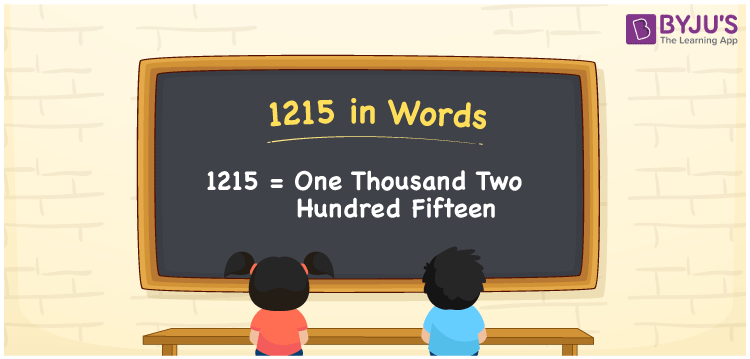# 1215 in Words

1215 in words is written as One thousand two hundred fifteen. In both the International System of Numerals and the Indian System of Numerals, 1215 is written as One thousand two hundred fifteen. The number 1215 is a Cardinal Number as it could represent some quantity. For example, “the area of this land is 1215 meters square”.

 1215 in Words One thousand two hundred fifteen One thousand two hundred fifteen in Number 1215

## 1215 in English Words

1215 in English words is read as “One thousand two hundred fifteen”.## How to Write 1215 in Words?

To write 1215 in words, we shall use the place value chart. In the place value chart, put 1 in the thousands, 2 in the hundreds, 1 in the tens, and 5 in the ones, respectively. Let us make a place value chart to write the number 1215 in words.

 Thousands Hundreds Tens Ones 1 2 1 5

Thus, we can write the expanded form as

1 × Thousand + 2 × Hundred + 1 × Ten + 5 × One

= 1 × 1000 + 2 × 100 + 1 × 10 + 5 × 1

= 1000 + 200 + 10 + 5

= 1215

= One thousand two hundred fifteen.

1215 is a natural number which is the successor of 1214 and the predecessor of 1216.

1215 in words – One thousand two hundred fifteen

• Is 1215 an odd number? – Yes
• Is 1215 an even number? – No
• Is 1215 a perfect square number? – No
• Is 1215 a perfect cube number? – No
• Is 1215 a prime number? – No
• Is 1215 a composite number? – Yes

## Frequently Asked Questions on 1215 in Words

Q1

### How to write 1215 in words?

1215 in words is written as One thousand two hundred fifteen.
Q2

### How to write 1215 in the International and Indian System of Numerals?

In both, the system of numerals, 1215 in words, is written as One thousand two hundred fifteen.
Q3

### How to write 1215 in a place value chart?

In the place value chart, write 1 in the thousands, 2 in the hundreds, 1 in the tens, and 5 in the ones, respectively.